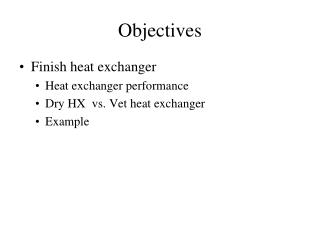DownloadDownload PresentationObjectives

# Objectives

Download Presentation## Objectives

- - - - - - - - - - - - - - - - - - - - - - - - - - - E N D - - - - - - - - - - - - - - - - - - - - - - - - - - -
##### Presentation Transcript

1. Objectives • Finish heat exchanger • Heat exchanger performance • Dry HX vs. Vet heat exchanger • Example

2. Heat exchangers Air-liquid Tube heat exchanger Air-air Plate heat exchanger

3. Fin Efficiency • Assume entire fin is at fin base temperature • Maximum possible heat transfer • Perfect fin • Efficiency is ratio of actual heat transfer to perfect case • Non-dimensional parameter

4. Heat exchanger performance (11.3) • NTU – absolute sizing (# of transfer units) • ε – relative sizing (effectiveness)

5. ExampleFollowing HW2 problem AHU M For the problem 9 HW assignment # 2 (process in AHU) calculate: a) Effectiveness of the cooling coil b) UoAo value for the CC Inlet water temperature into CC is coil is 45ºF OA CC CC (mcp)w RA tc,in=45ºF Qcc=…..Btu/h tM=81ºF tCC=55ºF

6. Summary • Calculate efficiency of extended surface • Add thermal resistances in series • If you know temperatures • Calculate R and P to get F, ε, NTU • Might be iterative • If you know ε, NTU • Calculate R,P and get F, temps

7. Analysis of Moist Coils • Redo fin theory • Energy balance on fin surface, water film, air Introduce Lewis Number • Digression – approximate enthalpy • Redo fin analysis for cooling/ dehumidification (t → h)

8. 1. Redo Fin Theory • Same result

9. 2. Energy and mass balances • Steady-state energy equation on air • Energy balance on water • Mass balance on water • Rewrite energy balance on water surface • Reintroduce hg0 (enthalpy of sat. water vapor at 0 °C or °F)

10. 3. Define heat-transfer coefficient for wet surface 4. Fin analysis for wet fins Heat conduction only occurs in y-direction through water film

11. Overview of Procedure • Same approach as for dry fin with addition of conduction through water film • Define “fictitous moist air enthalpy” define at water surface temperature • Define heat-transfer coefficient • Develop new governing equation

12. Overall Heat Transfer Coefficients • Very parallel procedure to dry coil problem • U-values now influenced by condensation • See Example 11.6 for details

13. Approximate Expression for Mean Enthalpy Difference h1 enthalpy of entering air stream h2 enthalpy of leaving air stream hs,R,1 fictitious enthalpy of saturated air at entering refrigerant temp. hs,R,2 fictitious enthalpy of saturated air at leaving refrigerant temp.

14. Wet Surface Heat Transfer • Approximate dry and wet wet-surface area and apply relevant equations Reynolds number changes – empirical relationships Questions: • Does a wet or a dry coil have higher or lower heat exchange? • Does a wet or a dry coil have higher or lower pressure drop?